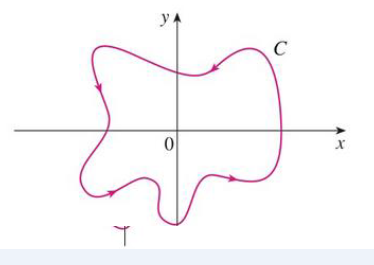Chapter 16, Problem 38RE

Chapter
Section
Textbook Problem

Let F(x, y) = ( 2 x 3 +   2 x y 2 − 2 y ) i + ( 2 y 3 +   2 x 2 y +   2 x ) j x 2 + y 2 Evaluate ∮C. F · dr, where C is shown in the figure.To determine

To evaluate: The value of CFdr , where F(x,y)=(2x3+2xy22y)i+(2y3+2x2y+2x)jx2+y2

Explanation

Given data:

F(x,y)=(2x3+2xy22y)i+(2y3+2x2y+2x)jx2+y2 (1)

Formula used:

Write the expression for Green’s Theorem.

CFdr=CFdr (2)

Write the trigonometric expression.

cos2x+sin2x=1

Consider C be the circle with center located at origin and radius a and D is the region bounded by C and C as shown in Figure 1.

D’s positively oriented boundary is represented as C(C) .

Find the value of CFdr .

CFdr=02πF(r(t))r(t)dt (3)

Write the expression of polar form representation.

x=rcost (4)

y=rsint (5)

Substitute a for r in equation (4),

x=acost

Substitute a for r in equation (5),

y=asint

Find the value of F(r(t)) .

Substitute r(t) for (x,y) , acost for x and asint for y in equation (1),

F(r(t))=[(2(acost)3+2(acost)(asint)22(asint))i+(2(asint)3+2(acost)2(asint)+2(acost))j](acost)2+(asint)2=[(2a3cos3t+2a3costsin2t2asint)i+(2a3sin3t+2a3sintcos2t+2acost)j]a2cos2t+a2sin2t

Consider the expression for r(t) as follows.

r(t)=xi+yj

Substitute acost for x and asint for y ,

r(t)=acosti+asintj

Differentiate equation with respect to t as follows.

r(t)=asinti+acostj

Substitute [(2a3cos3t+2a3costsin2t2asint)i+(2a3sin3t+2a3sintcos2t+2acost)j]a2cos2t+a2sin2t for F(r(t)) and asinti+acostj for r(t) in equation (3),

CFdr

Still sussing out bartleby?

Check out a sample textbook solution.

See a sample solution

The Solution to Your Study Problems

Bartleby provides explanations to thousands of textbook problems written by our experts, many with advanced degrees!

Get Started

Factor completely: 12x228x+15

Elementary Technical Mathematics

For f(x) = 10x2, f′(3) = _____. 10 20 30 60

Study Guide for Stewart's Single Variable Calculus: Early Transcendentals, 8th

Write as an iterated integral, where D is shown at the right.

Study Guide for Stewart's Multivariable Calculus, 8th